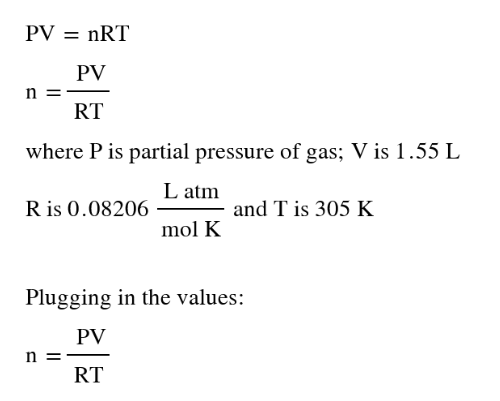# Problem: Carbon monoxide gas reacts with hydrogen gas to form methanol via the following reaction:CO(g) + 2 H2(g) → CH3OH(g)A 1.55 L reaction vessel, initially at 305 K, contains carbon monoxide gas at a partial pressure of 232 mmHg and hydrogen gas at a partial pressure of 376 mmHg .Identify the limiting reactant and determine the theoretical yield of methanol in grams.

🤓 Based on our data, we think this question is relevant for Professor Teets' class at UH.

###### FREE Expert Solution
• Calculate for the moles of each reactant gas and use stoichiometry to determine the moles and eventually mass of CH3OH
• Moles of reactant will be calculated using the conditions via the ideal gas equation
• Ideal gas equation will appear as:• Calculating the moles of reactant gases:###### Problem Details

Carbon monoxide gas reacts with hydrogen gas to form methanol via the following reaction:

CO(g) + 2 H2(g) → CH3OH(g)

A 1.55 L reaction vessel, initially at 305 K, contains carbon monoxide gas at a partial pressure of 232 mmHg and hydrogen gas at a partial pressure of 376 mmHg .

Identify the limiting reactant and determine the theoretical yield of methanol in grams.